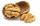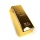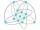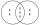# Plum

On the platter are plum. How many were there if its have to be able to share equally among 8,10 and 12 children?

n =  120

### Step-by-step explanation:Did you find an error or inaccuracy? Feel free to write us. Thank you!Tips to related online calculators
Do you want to calculate least common multiple two or more numbers?

## Related math problems and questions:

• NutsHow many we must have at least nuts if we can equally divide it to 10 children, 12 children or 15 children and any nut left?
• PlumsIn the bowl are plums. How many would be there if we can divide it equally among 8, 10 and 11 children?
• LCMWhat is the least common multiple of 5, 50, 14?
• The KingThe King wants to divide his sons equally. He has 42 rubies and 45 diamonds. How many sons and how will they share them?
• CherriesCherries in the bowl can be divided equally among 19 or 13 or 28 children. How many are the minimum cherries in the bowl?
• Lcm simpleFind least common multiple of this two numbers: 140 175.
• GroupA group of kids wanted to ride. When the children were divided into groups of 3 children, one remain. When divided into groups of 4 children, 1 remains. When divided into groups of 6 children, 1 remains. After divided into groups of 5 children, no one lef
• On Children'sOn Children's Day, the organizers bought 252 chewing gums, 396 candies and 108 lollipops. They want to make as many of the same packages as possible. Advise them what to put in each package and how many packages they can make this way.
• Apples and pearsMom divided 24 apples and 15 pears to children. Each child received the same number of apples and pears - same number as his siblings. How many apples (j=?) and pears (h=?) received each child?
• Lcm of three numbersWhat is the Lcm of 120 15 and 5
• JordanJordan wants to bring in cookie cakes to share with his 23 classmates for his birthday. His mom is willing to buy no more than 6 cookie cakes and Jordan must share the cakes equally between his classmates (and himself). Answer the questions to help Jordan
• Lesson exercisingThe lesson of physical education, pupils are first divided into three groups so that each has the same number. The they redistributed, but into six groups. And again, it was the same number of children in each group. Finally they divided into nine equal g
• LCMCommon multiple of three numbers is 3276. One number is in this number 63 times, second 7 times, third 9 times. What are the numbers?
• AthletesAthletes at the stadium could enter two-steps, three-steps, four-steps, five-steps, six-steps. There were more than 100 but less than 200. How many athletes were there?
• LCD 2The least common denominator of 2/5, 1/2, and 3/4
• Lcm 2Create the smallest possible number that is divisible by numbers 5,8,9,4,3
• Lcm to eliminate yIdentify the least common multiple that would eliminate the y-variable. 6x - 5y = -4 4x + 2y = 28